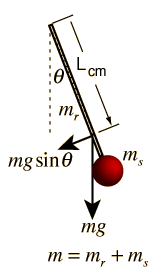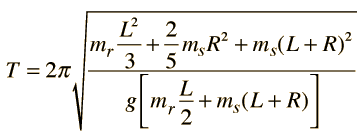# Combination Physical PendulumA physical pendulum composed of a uniform rod with a sphere on the end of it is an interesting example of a combination pendulum. By examining its moment of inertia, the period is determined to beFor a rod of length L = cm and mass mr = gm attached to a sphere of radius R=cmand mass ms = gm, with an acceleration of gravity g=m/s2, the period is T = s compared to a period of s for a simple pendulum for which length equals the distance to the center of mass of the sphere.
Index

Periodic motion concepts

 HyperPhysics***** Mechanics R Nave
Go Back